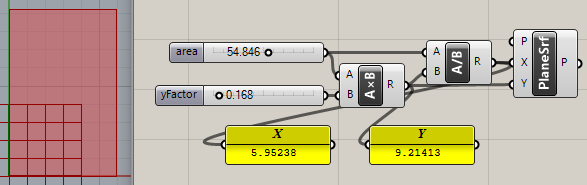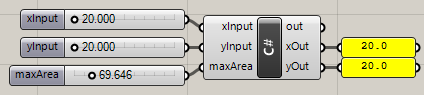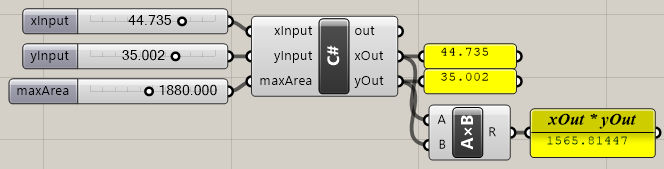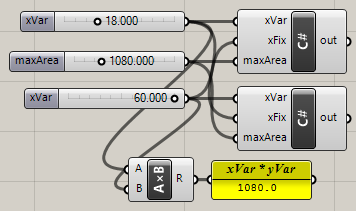# Dynamic rectangle sizing

Hello!

I’m trying to create a GH script (although help for developing a C# component would also be welcome!) to create a rectangle with variable sides that respond to a maximum area.

The behavior I’m looking for is to have the edges of the rectangle controlled by two sliders (one for x and one for y). If the area of the created rectangle >= a set max area, I want the sliders to “lock” and prevent the dimension being controlled to become larger.

You cannot lock the sliders, but you can certainly limit the values after they come out of the slider but before they are plugged into the rectangle component.

Do note that in Grasshopper there is no concept of which slider was moved last, whenever a slider is changed, a crisp new solution is started and the values from both sliders will be treated equally.

For example in this case you’d have two numbers w and h for the rectangle dimensions, and the area a = w \times h. Let us further say that the area is not supposed to exceed 100 m^2.

At some point in time w=20 and h=4.5 and all is well. Then the user drags the h slider and increases the value to h=5.1. Now the area would be 102m^2, so we have to limit the values. But which one? You don’t know which one was changed last, at least not without a lot of additional logic.

This isn’t quite the same thing, but might be interesting?

A single ‘Ratio’ slider is used to define the dimensions of the rectangle, which is constrained in both size and position (set by the ‘MD Slider’) by the “Limits” points.

See attached

Rectangle_based_on_area_V1.gh (117.3 KB)

Thanks Peter for this definition.
Why it is necessary to have FilletR? If we try 0 the rectangle disapears.
Thanks

Thanks for the help David! Confirms why I’m having so much trouble with this!

This is made having some fillet value (!=0) as a given. I’ll add a line or two more to work with no fillet. That said, I have another one that works with any polygon … but what was the def name ??

In fact, rather remove some.

Rectangle_based_on_area_V1A.gh (119.9 KB)rectangle_sizing_2018Jan25a.gh (5.6 KB)

1 Like

Here is a way with gh to control with X and Y side using scale.

ConstrainedArea.gh (11.6 KB)

3 Likes

Perfect PeterThanks
Thank you Joseph_Oster

And if you want to do for volume:

ConstrainedVolume.gh (12.4 KB)

I believe I picked this technique up from @andheum years ago on the old gh forum.

This is great but not exactly the behavior I was looking for!

I ended up using some C# cobbled together using info from this site:

The C# script has 3 inputs: xInput, yInput, and maxArea

The script changes the range of the sliders so that they can never go above the maxArea.
For example, for the xInput the maximum value it can be is the maxArea/yInput.

Here’s the code:

private void RunScript(double xInput, double yInput, double maxArea, ref object xOut, ref object yOut)
{

decimal xResult = (decimal) maxArea / (decimal) yInput;
decimal yResult = (decimal) maxArea / (decimal) xInput;

var xSliderInput = Component.Params.Input.Sources;
var xSlider = xSliderInput as Grasshopper.Kernel.Special.GH_NumberSlider;

var ySliderInput = Component.Params.Input.Sources;
var ySlider = ySliderInput as Grasshopper.Kernel.Special.GH_NumberSlider;

if(xSlider != null)
{
xSlider.Slider.Minimum = 20;
xSlider.Slider.Maximum = (decimal) xResult;
}

if(ySlider != null)
{
ySlider.Slider.Minimum = 20;
ySlider.Slider.Maximum = (decimal) yResult;
}

xOut = xInput;
yOut = yInput;


}

I’m new to C# so forgive any obvious errors!

Can you post a working example? Because this doesn’t work well at all for me:So ‘maxArea’ must be greater than 400? Still fails:Dtres_2018Jan26a.gh (6.5 KB)

Looks like the very problem @DavidRutten described:

I’m not a C# programmer but… here is a variation of that idea that sets the “other” slider’s value. It demonstrates a race condition that rarely reaches stability:Dtres_2018Jan26b.gh (7.1 KB)

  private void RunScript(double xVar, double xFix, double maxArea)
{
decimal result = (decimal) maxArea / (decimal) xVar;
var sliderInput = Component.Params.Input.Sources;
var slider = sliderInput as Grasshopper.Kernel.Special.GH_NumberSlider;
if(slider != null)
{
slider.Slider.Value = result;
}
}